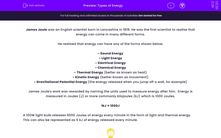# Simplify and Solve Simple Equations

In this worksheet, students will simplify like terms before solving simple equations.Key stage:  KS 3

Curriculum topic:   Algebra

Curriculum subtopic:   Solve Linear Equations (One Variable)

Popular topics:   Algebra worksheets

Difficulty level:#### Worksheet Overview

When we solve algebraic equations, our aim is to end up with one letter on one side of the equals sign and one number on the other.  This is the solution.

We do this by simplifying like terms if we can and then using inverse operations to undo things that get in the way, but remember that we must do the same thing to both sides.

Example

7b - 2b + 5b = -20

Simplify the left-hand side by combining like terms

10b = -20

Divide both sides by 10

10b ÷ 10 = -20 ÷ 10

Simplify

b = -2

Want a bit more help with this before you begin? Why not watch this short video?

### What is EdPlace?

We're your National Curriculum aligned online education content provider helping each child succeed in English, maths and science from year 1 to GCSE. With an EdPlace account you’ll be able to track and measure progress, helping each child achieve their best. We build confidence and attainment by personalising each child’s learning at a level that suits them.

Get started#### Popular Maths topics

••••